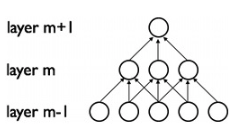# Convolutional Layer

Back to glossary

In deep learning, a convolutional neural network (CNN or ConvNet) is a class of deep neural networks, that are typically used to recognize patterns present in images but they are also used for spatial data analysis, computer vision, natural language processing, signal processing, and various other purposes The architecture of a Convolutional Network resembles the connectivity pattern of neurons in the Human Brain and was inspired by the organization of the Visual Cortex. This specific type of Artificial Neural Network gets its name from one of the most important operations in the network: convolution.

## What Is a Convolution?

Convolution is an orderly procedure where two sources of information are intertwined; it’s an operation that changes a function into something else. Convolutions have been used for a long time typically in image processing to blur and sharpen images, but also to perform other operations. (e.g. enhance edges and emboss) CNNs enforce a local connectivity pattern between neurons of adjacent layers.CNNs make use of filters (also known as kernels), to detect what features, such as edges, are present throughout an image. There are four main operations in a CNN:

• Convolution
• Non Linearity (ReLU)
• Pooling or Sub Sampling
• Classification (Fully Connected Layer)

The first layer of a Convolutional Neural Network is always a Convolutional Layer. Convolutional layers apply a convolution operation to the input, passing the result to the next layer. A convolution converts all the pixels in its receptive field into a single value. For example, if you would apply a convolution to an image, you will be decreasing the image size as well as bringing all the information in the field together into a single pixel. The final output of the convolutional layer is a vector. Based on the type of problem we need to solve and on the kind of features we are looking to learn, we can use different kinds of convolutions.

## The 2D Convolution Layer

The most common type of convolution that is used is the 2D convolution layer and is usually abbreviated as conv2D. A filter or a kernel in a conv2D layer “slides” over the 2D input data, performing an elementwise multiplication. As a result, it will be summing up the results into a single output pixel. The kernel will perform the same operation for every location it slides over, transforming a 2D matrix of features into a different 2D matrix of features.

## The Dilated or Atrous Convolution

This operation expands window size without increasing the number of weights by inserting zero-values into convolution kernels. Dilated or Atrous Convolutions can be used in real time applications and in applications where the processing power is less as the RAM requirements are less intensive.

## Separable Convolutions

There are two main types of separable convolutions: spatial separable convolutions, and depthwise separable convolutions. The spatial separable convolution deals primarily with the spatial dimensions of an image and kernel: the width and the height. Compared to spatial separable convolutions, depthwise separable convolutions work with kernels that cannot be “factored” into two smaller kernels. As a result, it is more frequently used.

## Transposed Convolutions

These types of comvolutions are also known as deconvolutions or fractionally strided convolutions. A transposed convolutional layer carries out a regular convolution but reverts its spatial transformation.